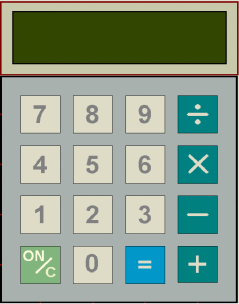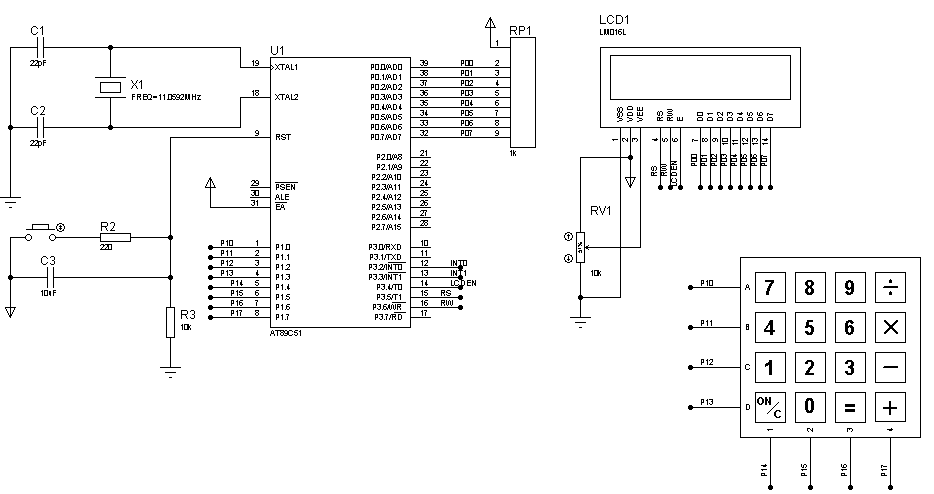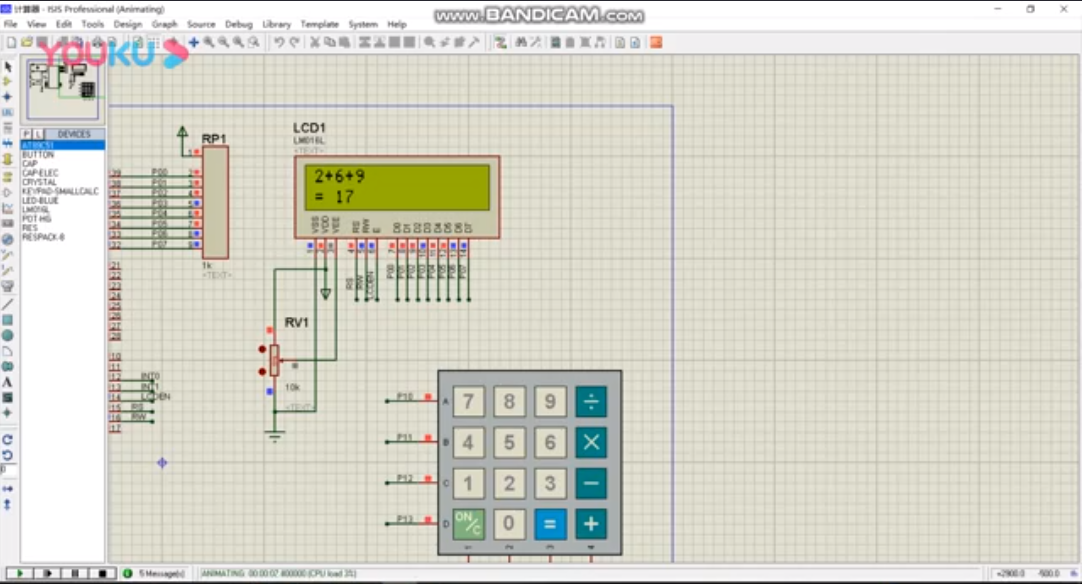• 用51单片机制作的简单计算器程序
千次阅读
2021-05-20 02:03:29

三、程序源代码

#include <reg51.h>#include <intrins.h>

#include <ctype.h>

#include <stdlib.h>

#define uchar unsigned char

#define uint unsigned int

uchar operand1, operand2;

uchar operator;

void delay(uint);

uchar keyscan();

void disp(void);

void buf(uint value);

uint compute(uint va1,uint va2,uchar optor);

uchar code table[] = {0xc0,0xf9,0xa4,0xb0,0x99,

0x92,0x82,0xf8,0x80,0x90,0xff};

uchar dbuf = {10,10,10,10,10,10,10,10};

void delay(uint z)

{

uint x,y;

for(x=z;x>0;x--)

for(y=110;y>0;y--);

}

uchar keyscan()

{

uchar skey;

P1 = 0xfe;

while((P1 & 0xf0) != 0xf0)

{

delay(3);

while((P1 & 0xf0) != 0xf0)

{

switch(P1)

{

case 0xee: skey = '7'; break;

case 0xde: skey = '8'; break;

case 0xbe: skey = '9'; break;

case 0x7e: skey = '/'; break;

default:   skey = '#';

}

while((P1 & 0xf0) != 0xf0)

;

}

}

P1 = 0xfd;

while((P1 & 0xf0) != 0xf0)

{

delay(3);

while((P1 & 0xf0) != 0xf0)

{

switch(P1)

{

case 0xed: skey = '4'; break;

case 0xdd: skey = '5'; break;

case 0xbd: skey = '6'; break;

case 0x7d: skey = '*'; break;

default:   skey = '#';

}

while((P1 & 0xf0) != 0xf0)

;

}

}

P1 = 0xfb;

while((P1 & 0xf0) != 0xf0)

{

delay(3);

while((P1 & 0xf0) != 0xf0)

{

switch(P1)

{

case 0xeb: skey = '1'; break;

case 0xdb: skey = '2'; break;

case 0xbb: skey = '3'; break;

case 0x7b: skey = '-'; break;

default: skey = '#';

}

while((P1 & 0xf0) != 0xf0)

;

}

}

P1 = 0xf7;

while((P1 & 0xf0) != 0xf0)

{

delay(3);

while((P1 & 0xf0) != 0xf0)

{

switch(P1)

{

case 0xe7: skey = '$'; break; case 0xd7: skey = '0'; break; case 0xb7: skey = '='; break; case 0x77: skey = '+'; break; default: skey = '#'; } while((P1 & 0xf0) != 0xf0) ; } } return skey; } void main() { uint value1, value2, value; uchar ckey, cut1 = 0, cut2 = 0; uchar operator; uchar i, bool = 0; init: buf(0); disp(); value = 0; cut1 = cut2 = 0; bool = 0; for(i = 0;i < 9;i++) { operand1[i] = '\0'; operand2[i] = '\0'; } while(1) { ckey = keyscan(); if(ckey != '#') { if(isdigit(ckey)) { switch(bool) { case 0: operand1[cut1] = ckey; operand1[cut1+1] = '\0'; value1 = atoi(operand1); cut1++; buf(value1); disp(); break; case 1: operand2[cut2] = ckey; operand2[cut2+1] = '\0'; value2 = atoi(operand2); cut2++; buf(value2); disp(); break; default: break; } } else if(ckey=='+'||ckey=='-'||ckey=='*'||ckey=='/') { bool = 1; operator = ckey; buf(0); dbuf = 10; disp(); } else if(ckey == '=') { value = compute(value1,value2,operator); buf(value); disp(); while(1) { ckey = keyscan(); if(ckey == '$')

goto init;

else

{

buf(value);

disp();

}

}

}

else if(ckey == '\$')

{    goto init;}

}

disp();

}

}

uint compute(uint va1,uint va2,uchar optor)

{

uint value;

switch(optor)

{

case '+' : value = va1+va2;    break;

case '-' : value = va1-va2;    break;

case '*' : value = va1*va2;    break;

case '/' : value = va1/va2;    break;

default :  break;

}

return value;

}

void buf(uint val)

{

uchar i;

if(val == 0)

{

dbuf = 0;

i = 6;

}

else

for(i = 7; val > 0; i--)

{

dbuf[i] = val % 10;

val /= 10;

}

for( ; i > 0; i--)

dbuf[i] = 10;

}

void disp(void)

{

uchar bsel, n;

bsel=0x01;

for(n=0;n<8;n++)

{

P2=bsel;

P0=table[dbuf[n]];

bsel=_crol_(bsel,1);

delay(3);

P0=0xff;

}

}

更多相关内容
• 基于c51单片机简单的计算器程序，简易计算器设计两位数加减运算 可实现三位数的加减乘除混合运算！将运算结果显示在数码管上！
• 可实现三位数的加减乘除混合运算！将运算结果显示在数码管上！
• 设计一个简易计算器，模拟常见计算器的加减乘除运算功能，通过1602液晶屏来显示数字、4*4的矩阵按键来模拟计算机的按键， 2、PROTEUS中设计的电路图 3、源代码 #include <reg51.h> #include <...

# 1、功能描述

设计一个简易计算器，模拟常见计算器的加减乘除运算功能，通过1602液晶屏来显示数字、4*4的矩阵按键来模拟计算机的按键，# 2、PROTEUS中设计的电路图# 3、源代码

#include <reg51.h>
#include <stdio.h>
#include <intrins.h>
#define u8  unsigned char
#define u16  unsigned char
sbit LCDEN=P3^4;
sbit RS=P3^5;
sbit RW=P3^6;
sbit BF=P0^7;
u8 code keyval[]="789/456*123-c0=+"; //按键对应的符号
u8 data1;
u8 k=0;
char  m={0};
double sum=0;
void delay(u16 x)	  //延时x毫秒
{
u16 i,j;
for(i=0;i<x;i++)
for(j=0;j<115;j++)
;
}

{
u8 i,row,temp;
u8 key=16;//按键号，初值设置为16，目的是：没有按键按下时返回16；
//若不设初值(默认值为0)，没有按键按下时，将返回0，会误认为0被按下
row=0xef; //从第一列开始
for(i=0;i<4;i++)
{
P1=0xff;
P1=row;	//第i列信号，对应列为低，其他全为高
row=_crol_(row,1); 	  //生成下一列信号
temp=P1; //读入扫描信号
temp=temp&0x0f; //屏蔽高4位列信号，只保留低4位行信号
if(temp!=0x0f)//有按键被按下，因为第i列某行有按键按下，则低4位中有一位为低
{
delay(20);  //延时去抖
temp=P1;
temp=temp&0x0f;
if(temp!=0x0f)   //再次确认有按键被按下
{
switch(temp)  //根据低4位行信号，判断哪个按键被按下
{
case 0x0e:key=0+i;break; //第i列第1行按键被按下
case 0x0d:key=4+i;break; //第i列第2行按键被按下
case 0x0b:key=8+i;break; //第i列第3行按键被按下
case 0x07:key=12+i;      //第i列第4行按键被按下
}

do
{
temp=P1;  	    //再次扫描按键
temp=temp&0x0f;
}while(temp!=0x0f); //等待按键释放
}
}
}
return(key);//扫面结束，返回按键值
}

unsigned char DectectBusyBit(void)//状态判断函数(忙/闲?)
{
bit result;
P0 = 0xff;	//读状态前先置高电平，防止误判
RS = 0;
delay(5);
RW = 1;
LCDEN = 1;
delay(5);
result=BF; //若LCM忙,则反复测试,在此处原地踏步；当LCM闲时，才往下继续
LCDEN = 0;
return result;
}

void WrComLCD(unsigned char ComVal)//写命令函数
{
while(DectectBusyBit()==1);         //先检测LCM是否空闲
RS = 0;
delay(1);
RW = 0;
LCDEN = 1;
P0 = ComVal;
delay(1);
LCDEN = 0;
}

void WrDatLCD(unsigned char DatVal)//写数据函数
{
while(DectectBusyBit()==1);
RS = 1;
delay(1);
RW = 0;
LCDEN = 1;
P0 = DatVal;
delay(1);
LCDEN = 0;
}

void LCD_Init(void)//1602初始化函数
{
WrComLCD(0x38);     // 功能设定:16*2行、5*7点阵、8位数据接口
WrComLCD(0x38);
WrComLCD(0x38);
//多次重复设定功能指令,因为LCD启动后并不知道使用的是4位数据接口还是8位的，所以开始时总是默认为4位
WrComLCD(0x01);    // 清屏
WrComLCD(0x06);    // 光标自增、屏幕不动
delay(1);	      // 延时，等待上面的指令生效，下面再显示，防止出现乱码
WrComLCD(0x0c);    // 开显示
}

void compute(){
u8 i,j=0,k,n=0;
char data3={0};
int sum1,data2={0};
sum=0;

for(i=0;data1[i]!='\0';i++){
if(data1[i]!='+' && data1[i]!='-' && data1[i]!='*' && data1[i]!='/'){
data2[j] =data2[j]*10+(data1[i]-'0');
}
else{
data3[n++] = data1[i];
j++;
}
}
for(i=0;i<n;i++){
if(i==0){
if(data3=='+')  sum = data2 + data2;
if(data3=='-')  sum = data2 - data2;
if(data3=='*')  sum = data2 * data2;
if(data3=='/')  sum = data2 / (double)data2;
}
if(i==1){
if(data3=='+')  sum = sum+data2;
if(data3=='-')  sum = sum-data2;
if(data3=='*')  sum = sum*data2;
if(data3=='/')  sum = sum/((float)data2);
}
if(i==2){
if(data3=='+')  sum = sum+data2;
if(data3=='-')  sum = sum-data2;
if(data3=='*')  sum = sum*data2;
if(data3=='/')  sum = sum/((float)data2);
}

}

//判断是小数输出还是整数输出
sum1 = sum;
if(sum1==sum){
sprintf(m,"%d",sum1);
}
else{
sprintf(m,"%f",sum);
}
//把结果输出出来
for(k=0;m[k]!='\0';k++){
WrDatLCD(m[k]);
}
}

void main()
{
u8 y;
LCD_Init();
delay(5);   //延时，等待初始化完成
WrDatLCD('0');

WrComLCD(0x80);	   //设置显示地址第一行第一位：0X00（0x80+0x00）
while(1){
if(y==12)  { k=0;WrComLCD(0x01);WrDatLCD('0'); WrComLCD(0x80);}   //清屏

if(y==14)  {
WrComLCD(0xC0); WrDatLCD(keyval[y]); WrDatLCD(' '); data1[k]='\0';compute();//调用出结果函数

}

if(y<16 && y!=12 && y!=14)
{
WrDatLCD(keyval[y]);
data1[k++]= keyval[y];
}
}

}

# 4、实验效果

由于不能上传视频，所以我将效果视频上传至优酷，请点击观看------->计算器效果视频现将工程源代码，以及电路图上传至百度云，供大家学习，如有问题，请留言

### 群号：245022761

展开全文• #include #include #include #define u8unsigned char#define u16unsigned charsbit LCDEN=P3^4;sbit RS=P3^5;sbit RW=P3^6;sbit BF=P0^7;u8 code keyval[]="789/456*123-c0=+"; //按键对应的符号u8 data1;...

#include

#include

#include

#define u8  unsigned char

#define u16  unsigned char

sbit LCDEN=P3^4;

sbit RS=P3^5;

sbit RW=P3^6;

sbit BF=P0^7;

u8 code keyval[]="789/456*123-c0=+"; //按键对应的符号

u8 data1;

u8 k=0;

char  m={0};

double sum=0;

void delay(u16 x)          //延时x毫秒

{

u16 i,j;

for(i=0;i

for(j=0;j<115;j++)

;

}

{

u8 i,row,temp;

u8 key=16;//按键号，初值设置为16，目的是：没有按键按下时返回16；

//若不设初值(默认值为0)，没有按键按下时，将返回0，会误认为0被按下

row=0xef; //从第一列开始

for(i=0;i<4;i++)

{

P1=0xff;

P1=row;        //第i列信号，对应列为低，其他全为高

row=_crol_(row,1);           //生成下一列信号

temp=P1; //读入扫描信号

temp=temp&0x0f; //屏蔽高4位列信号，只保留低4位行信号

if(temp!=0x0f)//有按键被按下，因为第i列某行有按键按下，则低4位中有一位为低

{

delay(20);  //延时去抖

temp=P1;

temp=temp&0x0f;

if(temp!=0x0f)   //再次确认有按键被按下

{

switch(temp)  //根据低4位行信号，判断哪个按键被按下

{

case 0x0e:key=0+i;break; //第i列第1行按键被按下

case 0x0d:key=4+i;break; //第i列第2行按键被按下

case 0x0b:key=8+i;break; //第i列第3行按键被按下

case 0x07:key=12+i;      //第i列第4行按键被按下

}

do

{

temp=P1;              //再次扫描按键

temp=temp&0x0f;

}while(temp!=0x0f); //等待按键释放

}

}

}

return(key);//扫面结束，返回按键值

}

unsigned char DectectBusyBit(void)//状态判断函数(忙/闲?)

{

bit result;

P0 = 0xff;        //读状态前先置高电平，防止误判

RS = 0;

delay(5);

RW = 1;

LCDEN = 1;

delay(5);

result=BF; //若LCM忙,则反复测试,在此处原地踏步；当LCM闲时，才往下继续

LCDEN = 0;

return result;

}

void WrComLCD(unsigned char ComVal)//写命令函数

{

while(DectectBusyBit()==1);         //先检测LCM是否空闲

RS = 0;

delay(1);

RW = 0;

LCDEN = 1;

P0 = ComVal;

delay(1);

LCDEN = 0;

}

void WrDatLCD(unsigned char DatVal)//写数据函数

{

while(DectectBusyBit()==1);

RS = 1;

delay(1);

RW = 0;

LCDEN = 1;

P0 = DatVal;

delay(1);

LCDEN = 0;

}

void LCD_Init(void)//1602初始化函数

{

WrComLCD(0x38);     // 功能设定:16*2行、5*7点阵、8位数据接口

WrComLCD(0x38);

WrComLCD(0x38);

//多次重复设定功能指令,因为LCD启动后并不知道使用的是4位数据接口还是8位的，所以开始时总是默认为4位

WrComLCD(0x01);    // 清屏

WrComLCD(0x06);    // 光标自增、屏幕不动

delay(1);              // 延时，等待上面的指令生效，下面再显示，防止出现乱码

WrComLCD(0x0c);    // 开显示

}

void compute(){

u8 i,j=0,k,n=0;

char data3={0};

int sum1,data2={0};

sum=0;

for(i=0;data1[i]!='\0';i++){

if(data1[i]!='+' && data1[i]!='-' && data1[i]!='*' && data1[i]!='/'){

data2[j] =data2[j]*10+(data1[i]-'0');

}

else{

data3[n++] = data1[i];

j++;

}

}

for(i=0;i

if(i==0){

if(data3=='+')  sum = data2 + data2;

if(data3=='-')  sum = data2 - data2;

if(data3=='*')  sum = data2 * data2;

if(data3=='/')  sum = data2 / (double)data2;

}

if(i==1){

if(data3=='+')  sum = sum+data2;

if(data3=='-')  sum = sum-data2;

if(data3=='*')  sum = sum*data2;

if(data3=='/')  sum = sum/((float)data2);

}

if(i==2){

if(data3=='+')  sum = sum+data2;

if(data3=='-')  sum = sum-data2;

if(data3=='*')  sum = sum*data2;

if(data3=='/')  sum = sum/((float)data2);

}

}

//判断是小数输出还是整数输出

sum1 = sum;

if(sum1==sum){

sprintf(m,"%d",sum1);

}

else{

sprintf(m,"%f",sum);

}

//把结果输出出来

for(k=0;m[k]!='\0';k++){

WrDatLCD(m[k]);

}

}

void main()

{

u8 y;

LCD_Init();

delay(5);   //延时，等待初始化完成

WrDatLCD('0');

WrComLCD(0x80);           //设置显示地址第一行第一位：0X00(0x80+0x00)

while(1){

if(y==12)  { k=0;WrComLCD(0x01);WrDatLCD('0'); WrComLCD(0x80);}   //清屏

if(y==14)  {

WrComLCD(0xC0); WrDatLCD(keyval[y]); WrDatLCD(' '); data1[k]='\0';compute();//调用出结果函数

}

if(y<16 && y!=12 && y!=14)

{

WrDatLCD(keyval[y]);

data1[k++]= keyval[y];

}

}

}

展开全文• 设计了一个以宏晶STC89C52RC为核心的8位整数运算的计算器。首先提出了设计要求,然后进行方案论证、器件选型及硬件原理图的设计,最后给出了8位整数的加、减、乘、除运算程序
• 《基于C51单片机简易计算器的课程设计.doc》由会员分享，可免费在线阅读全文，更多与《基于C51单片机简易计算器的课程设计(最终版)》相关文档资源请在帮帮文库(www.woc88.com)数亿文档库存里搜索。1、位七段码，H...

《基于C51单片机简易计算器的课程设计.doc》由会员分享，可免费在线阅读全文，更多与《基于C51单片机简易计算器的课程设计(最终版)》相关文档资源请在帮帮文库(www.woc88.com)数亿文档库存里搜索。

1、位七段码，H单元存放数值的百位七段码，H单元存放数值的千位七段码。本方案设计中由P口输出字形码，P口输出字位码。先将存放于H单元的数值个位七段码由P口输出，同时P口输出使数值个位显示数码管点亮的字位码。由于采用的是共阴数码管，所以只有该位数码管对应的P为，其他位PP位，点亮延时MS。然后P口输出数值十位七段码，P位，数值十位数码管点亮，延时MS。接着P口输出数值百位七段码，P为，数值百的点亮时间为～ms，由于人的视觉暂留现象及发光二极体的余辉效应，尽管实际上各位数码管并非同时点亮，但只要扫描的速度足够快，给人的印象就是一组稳定的显示资料，不会有闪烁感，动态显示的效果和静态显示是一样的，能够节省大量的IO埠，而且功耗更低。综上所述我们在本设计方案中选用了动态显示。位LED显示的程序框图如图所示：图LED显示流程图主程序设计主程序进行程序中用到的一些存储单元的初始化，数值显示和*键盘扫描。首先，进行存储单元初始化，给数码管显示单元HH赋予“”字形数据，将数值计数单元，存储单元，HH,HH,H,H,AH,BH,CH,赋予初值零。之后，调用键盘扫描子

2、amnumgt=amamnumlt=)dislay(num)；el使用的标准键盘就是编码键盘。在智能仪器中，使用并行接口芯片或串行接口芯片HD均可以组成编码键盘，同时还可以兼顾数码管的显示驱动，其相关的接口电路和接口软件均可以在相关资料中得到。当系统功能比较复杂，案件数量很多时，采用编码键盘可以简化软件设计。但大多数智能仪器和电子产品的按键数目都不很多(个以内)，为了降低成本和简化电路通常采用非编码键盘。非编码键盘的电路由设计者根据需要自己决定，按键信息通过接口软件来获取。本课题需要个按键，故选择非编码键盘，为了减少所占用的端口，由P口采用*矩阵式键盘。图键盘样式输出电路设计数码管按段数分为七段数码管和八段数码管，八段数码管比七段数码管多一个发光二极管单元(多一个小数点显示)；按能显示多少个“”可分为位、位、位等等数码管；按发光二极管单元连接方式分为共阳极数码管和共阴极数码管。共阳级数码管是指将所有发光二极管的阳极接到一起形成公共阳极(COM)的数码管。共阳级数码管在应用时应将公共极COM接到+V，当某一字段发光二极管的阴极为低电平时，相应字

3、段就点亮。当某一字段的阴极为高电平时，对应的字段就不亮。。共阴数码管是指将所有发光二极管的阴极接到一起形成公共阴极(COM)的数码管。共阴数码管在应用时应将公共极COM接到地线GND上，当某一字段发光二极管的阳极为高电平时，相应字段就点亮。当某一字段的阳极为低电平时，对应的字段就不亮。LED显示器由七段发光二极管组成，排列成字形状，因此也成为七段LED显示器，器排列形状如下图所示：图LED段码为了显示数字或符号，要为LED显示器提供代码，即字形代码。七段发光二极管，再加上一个小数点位，共计段，因此提供的字形代码的长度正好是一个字节。简易计算器用到的数字~的共阴极字形代码如下表所示：显示字型gfedcBa段码fhhbhfhhdhdhhfhfh表~七段数码管共阴级字形代码由于数值单元存放的是二进制数，而我们大家熟悉的是十进制数，所以应将数值单元中的二进制数字转换为十进制数字，即BCD码。要通过数码管显示出当前的数值，还必须将BCD码进一步转换为七段码，转换的最终结果数据存放于显示缓冲区HH单元中，其中H单元存放数值的个位七段码，H单元存放数值的十

4、=)按下ONC键，清零{flag_fuhao=；shu=shu=fuhao=flag_shu=newkey=；}elseif(key_shu==amamflag_shu==amamfuhao)按下quot=quot{switch(fuhao){case:shu=shu+shu；eak；case:shu=shushu；eak；case:shu=shu*shu；eak；case:shu=shushu；eak；}fuhao=；flag_fuhao=；}elseif((key_shugt=)amam(key_shu=)amam(key_shult=))按下运算符{flag_fuhao=；if(flag_shu==){if(fuhao==){shu=shu；shu=；fuhao=key_shu；flag_shu=；}else{switch(fuhao){case:shu=shu+shu；eak；case:shu=shushu；eak；case:shu=shu*shu；eak；case:shu=shushu；eak；}shu=；fuhao=key_shu

5、。最后P口输出数值千位七段码，P为，数值千位数码管点亮，延时MS。发光二极管LED是单片机应用系统中的一种简单而最常用的输出设备，其在系统中的主要作用是显示单片机的输出数据、状态等。因而作为典型的外围器件，LED显示单元是反映系统输出和操作输入的有效元器件。LED具备数字接口可以方便的和单片机引脚连接；它的优点是价格低，寿命长，对电压电流的要求低以及容易实现多路等，因而在单片机应用系统中获得了广泛的应用，所以在此设计中我首先选用了LED作为显示器件。如图所示：图数码显示管程序设计本方案中的程序设计采用了模块化设计，各部分程序都分别进行独立的设计，最后主程序通过调用各模块程序来运行，编程中所使用的语言全部都是C语言，可以利用keil软件进行灵活的编译，编译完成后也可生成HEX文件，利用ISP编程软件通过串口写到单片机中。本方案程序设计中部分包括主程序模块、液晶显示模块、功能按键和控制输出等部分。下面仅仅叙述了各部分程序设计的基本思想和流程图，详细程序请参阅附录。读键输入程序流程图为了实现键盘的数据输入功能和命令处理功能，每个键都有其处理子程序，

6、，通过我的不懈努力，在动手方面，我会得到提高。这一点，我坚信。在此次的课程设计中我最大的体会就是进一步认识到了理论联系实践的重要性。一份耕耘，一份收获。通过这段时间的设计，让我明白科学的思维方法和学习方法是多么重要，只有这样才能够有很高的效率，才能够让自己的工作更完美。总而言之，此次毕业设计让我学到了好多平时在课堂上学不到的东西，增加了我的知识运用能力，增强我的实际操作能力。谢谢老师给我们提供这么好的机会，为我们之后走向社会奠定了一个好的基础。在我做课程设计的整个过程中，无论是在设计的选题、构思和资料的收集方面，还是在设计的研究方法以及成文定稿方面，或是在论文的写作过程中，都得到了许多同学的宝贵建议，在此一并致以诚挚的谢意。感谢所有关心、支持、帮助过我的同学。元件清单元件数量单价(元)M晶振STCC芯片轻触按键排阻四位一体公阴数码管电阻若干杜邦线排针电容若干总计附录简易计算器源程序includedefineucharunsignedchardefineuintunsignedintdefineduanP数码管显示段选定义defineweiP数

7、较符合人们的日常思维，缺点是一级的程序错误会对整个程序产生影响。功能和操作：加减乘除运算和显示。A：上电后，屏幕初始化，按下“ONC”键。B:计算。按下数字键，屏幕显示要运算的第一个数字，再按下符号键，然后再按下数字键，屏幕显示要运算的第二个数字，最后按下“﹦”号键，屏幕上显示出计算结果。C:如果要再次计算，可以按下“ONC”键清零，或者按下单片机的复位键，重新初始化。硬件联系图如下图：硬件连线图结论课程设计是培养学生运用所学的专业知识，发现，提出，分析和解决实际问题，锻炼实际动手能力的重要环节，是对学生实际工作能力的具体训练和考察，随着科学技术日新月异的发展，单片机已经成为当今计算机应用中空前活跃的领域，在生活中可以说遍布我们生活之中。这次设计进一步端正了我的学习态度，学会了实事求是，严谨的作风，对自己要严格要求。急于求成是不好的，通过此次毕业设计我深有体会。如果省略了那些必要的步骤，急于求成，不仅会浪费时间，还会适得其反。我觉得动手之前，应该有清楚的步骤，这一步是很重要的。就目前来说，我的动手能力虽然还有差距，但我知道数码管点亮，延时MS

8、in(){flag=；标志关机while(){if(keyscan()==)开机检测{flag=；标志开机shu=shu=fuhao=flag_shu=newkey=；初始化变量while(flag){if(!flag_fuhao)dislay(shu)；elsedislay(shu)；key_shu=keyscan()；if(newkey==)sedislay(shu)；}returnnum；}}key=xfb；if(key!=xfb){delay()；if(key!=xfb){switch(key){casexeb:num=；eak；casexdb:num=；eak；casexbb:num=；eak；casexb:num=；eak；}newkey=；delay()；while(key!=xfb){if(flag)if(!shuamamnumgt=amamnumlt=)dislay(num)；elsedislay(shu)；}returnnum；}}key=xf；if(key!=xf){delay()；if(key!=xf){switch(

9、；}}elseif(flag_shu==)fuhao=key_shu；}newkey=；}}}}}键盘扫描ucharkeyscan(){key=xfe；if(key!=xfe){delay()；if(key!=xfe){switch(key){casexee:num=；eak；casexde:num=；eak；casexbe:num=；eak；casexe:num=；eak；quotquot}newkey=；delay()；while(key!=xfe){if(flag)if(!shuamamnumgt=amamnumlt=)dislay(num)；elsedislay(shu)；}returnnum；}}key=xfd；if(key!=xfd){delay()；if(key!=xfd){switch(key){casexed:num=；eak；casexdd:num=；eak；casexbd:num=；eak；casexd:num=；eak；*}newkey=；delay()；while(key!=xfd){if(flag)if(!shua

10、码管显示位选定义definekeyP键盘接口定义sbitOFF=P^；关机键定义unsignedlongintshu,shu；进行运算的两个变量数ucharnum；键盘扫描返回值charflag,flag_shu,flag_fuhao,fuhao,newkey；flag开机标志新按键标志，fuhao运算符charkey_shu；按键值unsignedcharcodeWela[]={xdf,xef,xf,xfb,xfd,xfe}；六位数码管的位选unsignedcharcodeDuan[]={xf,x,xb,xf,x,xd,xd,x,xf,xf,x}；无显示共阴极数码管ucharkeyscan()；键盘扫描函数voiddislay(unsignedlongint)；数码管显示函数voiddelay(uinti)延时函数{while(i)；}***********************************************主函数************************************************voidma

11、序，和数码管显示数据转换程序，数码管动态显示子程序。主程序不断进行键盘扫描，数码管显示数据转换子程序和动态显示子程序。首先初始化参数，送LED低位显示“”，功能键(“+”、“”、“*”、“”、“+”)位不显示。然后扫描键盘看是否有键输入，若有，读取键码。判断键码是数字键、清零键还是“+”、“”、“*”“”，是数值键则送LED显示并保存数值，是清零键则做清零处理，是功能键则又判断是“=”还是运算键，若是“=”则计算最后结果并送LED显示，若是运算键则保存相对运算程序的首地址。运算主程序框图如所示：图运算主程序框图仿真与调试在程序设计方法上，模块化程序设计是单片机应用中最常用的程序设计方法。设计的中心思想是把一个复杂应用程序按整体功能划分成若干相对独立的程序模块，各模块可以进行单独的设计、编程和调试，然后组合起来。这种方法便于设计和调试，容易实现多个程序共存，但各个模块之间的连接有一定的难度。根据需要我们可以采取自上而下的程序设计方法，此方法先从主程序开始设计，然后再编制各从属程序和子程序，层层细化逐步完成，最终完成一个复杂程序的设计。这种方法比

12、ey){casexe:num=；eak；ONCcasexd:num=；eak；casexb:num=；eak；=casex:num=；eak；+}newkey=；delay()；while(key!=xf){if(flag)if(!shuamamnumgt=amamnum)dis_flag=；elseif(dis_shugt)dis_flag=；elseif(dis_shugt)dis_flag=；elseif(dis_shugt)dis_flag=；elseif(dis_shugt)dis_flag=；elsedis_flag=；for(dis_aa=；dis_aaltdis_flag；dis_aa++){dis_data[dis_aa]=dis_shu%；dis_shu=dis_shu；}数码管段选for(dis_aa=；dis_aaltdis_flag；dis_aa++){duan=Duan[dis_data[dis_aa]]；wei=Wela[dis_aa]；delay()；wei=xff；}数码管位选}新键值{if(key_shu=

展开全文• 基于STC89C51单片机的实用计算器设计.pdf
• 本实验设计计算器可完成加减乘除四则运算，压缩包中含有纤细资料，包括protuse仿真，PCB板图设计和源代码。程序简单易懂。
• 基于单片机C51计算器源代码（附图）， 包含加减乘除以及有浮点运算的简易计算器
• 电路的硬件设计 53.1 系统框图 53.2 微处理器的选择 53.3 电源模块 63.4 AT89C51单片机参数 63.5 键盘的设计 83.6 复位电路的设计 93.7 振荡电路的设计 93.8 数码显示及驱动电路 94. 电路调试和结论 114.1 具体电路...
• 基于51单片机的简易计算器设计。本设计就是以单片机的基本语言汇编语言来进行软件设计，软件的设计采用模块化结构，使程序设计的逻辑关系更加简洁明了。使硬件在软件的控制下协调运作。单片机
• 按键说明56--515数字0--9的输入s16清零S17等于s18--s21加减乘除程序功能：本程序为简易计算器。可以算整数且正数类型的运算。但是暂时没有实现其连算功能，故在每次运算之后，请按下清零键。
• 基于C51单片机的多功能计算器全套资料(原理图+仿真+ 软件源程序+文档资料) 本设计是以STC89C52单片机为核心的计算器模拟系统设计，输入采用5×8矩阵键盘，可以进行加、减、乘、除等十几种数字运算，同时支持括号的...
• 实战型C51单片机程序例程软件源码20例合集： 10、LED流水+定时器.zip 11、LCD1602滚动显示.zip 12、LCD1602显示红外值.zip 13、LCD1602显示矩阵按键键值.zip 14、LCD显示温度+串口接收温度.zip 15、按键控制蜂鸣器....
• c51单片机简单计算器 提示：文章写完后，目录可以自动生成，如何生成可参考右边的帮助文档 文章目录c51单片机简单计算器前言代码缺点 前言 本文代码在下文博主给出的代码基础上进行了修改，关于硬件的介绍可以参考...51单片机 c语言
• #include <reg52.h> #include "math.h" #define GPIO_KEY P1 typedef unsigned int u16; typedef unsigned char u8; sbit LSA=P2^2; sbit LSB=P2^3;...u8 DisplayData={0x00,0x00,0x00
• 基于51单片机的计算器设计程序代码(汇编)DBUFEQU 30HTEMP EQU 40HYJ EQU50H ;结果存放YJ1 EQU51H ;中间结果存放GONG EQU52H ;功能键存放ORG 00HSTART:MOV R3,#0 ;初始化显示为空MOV GONG,#0MOV ...
• 51单片机：计算器（包含小数计算） 编写语言：C 运行情况：完美运行，尚未发现bug 备注：同普通实现小数计算程序不一样（浮点型数据计算存在精度丢失的情况），本程序全部采用整形进行计算，对小数点进行记录，最后...
• C51单片机设计C语言实例（400例）合集 大量设计实例，新手必备C51源码, 1-IO输出-点亮1个LED灯方法1 10-LED循环左移 100-24c02记忆开机次数 101-24c02存储上次使用中状态 102-DS1302 时钟原理 103-DS1302可调时钟 ...
• 设计是以51单片机为核心的计算器模拟系统设计，输入采用4×4矩阵键盘，可以进行加、减、乘、除4位带符号数字运算，并在LCD1602上显示操作过程。。 主要由51单片机+最小系统+LCD1602液晶显示模块+4*4矩阵按键电路；...
• PIC，EMC，A51，C51，数组。 你可以通过两种方式生动LED段码数据： 1、自动方式 根据你选择的生成数据区内容自动连续生成 数据。 2、手动方式 你可以直接用鼠标修改LED图标区的图形， 程序将按你的LED图标区的...
• ## 基于Proteus的C51远程计算器

千次阅读 热门讨论 2019-11-24 17:31:25
基于Proteus的C51远程计算器 一、具体要求： 1、 主机的矩阵键盘输入数和运算符号，从机显示运算结果 2、 主从单片机串行通信系统，并在 LCD 上显示运算内容及结果 二、实验要点： 1、按键扫描 2、串口通信 3...Proteus
• //倘若K=0，那么每隔三位就有一个小数点，计算器上的逗号就是这个原理。 367 }368 }369 if(c>99990000)370 {371 for(ei=0;ei;ei++)372 {373 lcdwrd(dat2[ei]);374 }375 }376 if(0<(a/b))377 {378 //lcdwrd(0x2e); ...
• ## 基于51单片机的计算器设计

万次阅读 多人点赞 2019-04-22 16:05:21
本次设计设计一个简易计算器，能够进行多位的加减乘除运算。它主要由51单片机的数码管，键盘等模块组成。本计算器是将键盘输入信息经处理通过缓存，送入数码管显示，数码管采用动态扫描方式，计算功能通过软件实现...51单片机 C语言
• 单片机简易计算器全套（源程序、hex文件、Proteus仿真、设计报告） 包含8套不同的简易计算器的代码。
•  这是嵌入式课程的一个小作业，用C51单片机，实现了0-255内的简易四则运算，暂不支持负数、溢出等特殊情况的处理。 【关键点】  1、计算器用R5、R6、R7三位显示，段码为0，全暗，段码为0xff，全亮。  2、...汇编 单片机...